• 多层前馈神经网络
千次阅读
2020-11-13 22:16:00

# 【神经网络】多层前馈神经网络 BP (附MATLAB程序)

## 1、数据预处理

​  ​ ​在训练神经网络前一般需要对数据进行预处理，一种重要的预处理手段是归一化处理。下面简要介绍归一化处理的原理与方法。

### 1.1 什么是归一化？

​  ​数据归一化是将数据映射到[0,1]或[-1,1]区间或更小的区间，比如(0.1,0.9)

### 1.2 为什么要归一化处理？

​​  ​(1) 输入数据的单位不一样，有些数据的范围可能特别大，导致的结果是神经网络收敛慢、训练时间长。

​​  ​(2)数据范围大的输入在模式分类中的作用可能会偏大，而数据范围小的输入作用就可能会偏小。

​​  ​(3)由于神经网络输出层的激活函数的值域是有限制的，因此需要将网络训练的目标数据映射到激活函数的值域。例如神经网络的输出层若采用S形激活函数，由于S形函数的值域限制在(0,1)，也就是说神经网络的输出只能限制在(0,1)，所以训练数据的输出就要归一化到[0,1]区间。

​​  ​(4)S形激活函数在(0,1)区间以外区域很平缓，区分度太小。例如S形函数f(X)在参数a=1时，f(100)与f(5)只相差0.0067。

### 1.3 归一化算法

数据归一化应该针对属性，而不是针对每条数据，针对每条数据是完全没有意义的，因为只是等比例缩放，对之后的分类没有任何作用。这是我遇到的 第一个坑。
​​  ​一种简单而快速的归一化算法是线性转换算法。线性转换算法常见有两种形式：

• y = ( x - min )/( max - min )

​​  ​其中min为x的最小值，max为x的最大值，输入向量为x，归一化后的输出向量为y 。上式将数据归一化到 [ 0 , 1 ]区间，当激活函数采用S形函数时（值域为(0,1)）时这条式子适用。

• y = 2 * ( x - min ) / ( max - min ) - 1
​​  ​这条公式将数据归一化到 [ -1 , 1 ] 区间。当激活函数采用双极S形函数（值域为(-1,1)）时这条式子适用。

### 1.4 Matlab数据归一化处理函数

训练集和测试集归一化的方法应该相同，但是在具体实验过程中，脑子发生了短路，对训练集的归一化是针对属性的，但是测试集却针对了数据，这是我遇到的 第二个坑。

​​  ​Matlab中归一化处理数据可以采用premnmx ， postmnmx ， tramnmx 这3个函数。

#### 1.4.1 premnmx

语法：[pn,minp,maxp,tn,mint,maxt] = premnmx(p,t)

参数

​​  ​pn： p矩阵按行归一化后的矩阵

​​  ​minp，maxp：p矩阵每一行的最小值，最大值

​​  ​tn：t矩阵按行归一化后的矩阵

​​  ​mint，maxt：t矩阵每一行的最小值，最大值

作用：将矩阵p，t归一化到[-1,1] ，主要用于归一化处理训练数据集。

#### 1.4.2 tramnmx

语法：[pn] = tramnmx(p,minp,maxp)

参数

​​  ​minp，maxp：premnmx函数计算的矩阵的最小，最大值

​​  ​pn：归一化后的矩阵

作用：主要用于归一化处理待分类的输入数据。

#### 1.4.3 postmnmx

语法： [p,t] = postmnmx(pn,minp,maxp,tn,mint,maxt)

参数

​​  ​minp，maxp：premnmx函数计算的p矩阵每行的最小值，最大值

​​  ​mint，maxt：premnmx函数计算的t矩阵每行的最小值，最大值

作用：将矩阵pn，tn映射回归一化处理前的范围。postmnmx函数主要用于将神经网络的输出结果映射回归一化前的数据范围。

## 2、 使用Matlab实现神经网络

使用Matlab建立前馈神经网络主要会使用到下面3个函数：

​​  ​newff ：前馈网络创建函数

​​  ​train：训练一个神经网络

​​  ​sim ：使用网络进行仿真

下面简要介绍这3个函数的用法。

### 2.1 newff函数

#### 2.1.1 newff函数语法

​​  ​newff函数参数列表有很多的可选参数，具体可以参考Matlab的帮助文档，这里介绍newff函数的一种简单的形式。

语法：net = newff ( A, B, {C} ,‘trainFun’)

参数

​​  ​A：一个n×2的矩阵，第i行元素为输入信号xi的最小值和最大值；

​​  ​B：一个k维行向量，其元素为网络中各层节点数；

​​  ​C：一个k维字符串行向量，每一分量为对应层神经元的激活函数

​​  ​trainFun ：为学习规则采用的训练算法

#### 2.1.2 常用的激活函数

​​  ​常用的激活函数有：

a) 线性函数 (Linear transfer function)

​ ​​  ​ f(x) = x 该函数的字符串为’purelin’

b) 对数S形转移函数( Logarithmic sigmoid transfer function )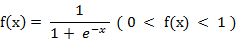该函数的字符串为’logsig’

c) 双曲正切S形函数 (Hyperbolic tangent sigmoid transfer function )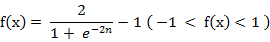​​  ​也就是上面所提到的双极S形函数, 该函数的字符串为’tansig’

​​  ​Matlab的安装目录下的toolbox\nnet\nnet\nntransfer子目录中有所有激活函数的定义说明。

#### 2.1.3 常见的训练函数

常见的训练函数有：

​​  ​traingd ：梯度下降BP训练函数(Gradient descent backpropagation)

​​  ​traingdx ：梯度下降自适应学习率训练函数

#### 2.1.4 网络配置参数

一些重要的网络配置参数如下：

​​  ​net.trainparam.goal ：神经网络训练的目标误差

​​  ​net.trainparam.show ： 显示中间结果的周期

​​  ​net.trainparam.epochs 　：最大迭代次数

​​  ​net.trainParam.lr ： 学习率

### 2.2 train函数

​​  ​网络训练学习函数

语法：[ net, tr, Y1, E ] = train( net, X, Y )

参数

​​  ​X：网络实际输入

​​  ​Y：网络应有输出

​​  ​tr：训练跟踪信息

​​  ​Y1：网络实际输出

​​  ​E：误差矩阵

### 2.3 sim函数

语法：Y=sim(net,X)

参数

​​  ​net：网络

​​  ​X：输入给网络的Ｋ×N矩阵，其中K为网络输入个数，N为数据样本数

​​  ​Y：输出矩阵Q×N，其中Q为网络输出个数

## 3、参数设置对神经网络性能的影响

​​  ​我在实验中通过调整隐含层节点数，选择不通过的激活函数，设定不同的学习率

### 3.1 隐含层节点个数

​​  ​隐含层节点的个数对于识别率的影响并不大，但是节点个数过多会增加运算量，使得训练较慢。

### 3.2 激活函数的选择

​​  ​ 激活函数无论对于识别率或收敛速度都有显著的影响。在逼近高次曲线时，S形函数精度比线性函数要高得多，但计算量也要大得多。

### 3.3 学习率的选择

​​  ​学习率影响着网络收敛的速度，以及网络能否收敛。学习率设置偏小可以保证网络收敛，但是收敛较慢。相反，学习率设置偏大则有可能使网络训练不收敛，影响识别效果。

## 4、Matlab BP神经网络实例

​​  ​我将Iris数据集分为2组，每组各75个样本，每组中每种花各有25个样本。其中一组作为以上程序的训练样本，另外一组作为检验样本。为了方便训练，将3类花分别编号为1，2，3 。

​​  ​使用这些数据训练一个4输入（分别对应4个特征），3输出（分别对应该样本属于某一品种的可能性大小）的前向网络。

​​  Matlab源码：BP神经网络实例神经网络 Algorithm
更多相关内容
• 此代码使用一阶随机梯度下降优化多层前馈神经网络。 它将网络输出为一个结构，然后可以在新数据上进行测试。matlab
• 构建一个至少含有1-2 层隐藏层的神经网络模型，解决手写的0-9 十个手写 数字的识别问题。神经网络模型构建过程中需要注意的几点： （1）数据集采用MNIST 阿拉伯数字手写体数据集。 （2）模型输入层的节点个数的设计...python
• 不同的层次结构会产出不同类型神经网络，比如前馈神经网络和递归神经网络。 神经元 神经元是神经网络最重要的组成部分，下图为一个神经元的示意图，箭头的方向就是数据运行的方向。为神经元接受的输入向量a，为...

神经网络是将许多个神经元按一定的层次结构连接起来的网络结构。不同的层次结构会产出不同类型神经网络，比如前馈神经网络和递归神经网络。

神经元

神经元是神经网络最重要的组成部分，下图为一个神经元的示意图，箭头的方向就是数据运行的方向。为神经元接受的输入向量a，为输入向量各个分量的权重w；b为偏置项bias，该条连接的输入固定为1。它可以让建模能力更强，更灵活。红色圆圈内为一个神经元处理信息的过程，里面又包括了SUM和f两部分。SUM为一个线性函数，将输入向量和权重向量的内积，输出的结果再传入激活函数f，最后输出结果t。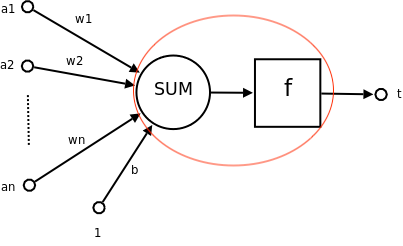一个神经元的功能是求得输入向量与权重向量的内积后，经过一个非线性函数也就是激活函数得到一个标量结果。

它会把一个n维向量空间用一个超平面分割成两部分，给定一个输入向量，神经元可以判断出这个向量位于超平面的哪一边。所以一个神经元就相当于做了一个二分类。(做回归任务的时候，神经元也相当于一个二分类吗？)

我们主要讲多层前馈神经网络。

多层前馈神经网络

多层前馈神经网络是由输入层，若干个隐藏层和输出层组成的全连接网络，不存在环或回路。。每一层都由多个神经元组成，神经元和输入向量全连接，但是神经元和神经元之间没有连接，也不存在跨层连接。每一层的输出结果会作为下一层的输入向量，比如hidden layer1的输出向量为hidden layer2的输入向量。输入层不会对数据进行处理，只是代表整个神经网络最开始的输入向量，也就是样本的特征向量。神经网络的输入为向量，最后的输出也是向量。输出向量的维度取决于输出层神经元的个数。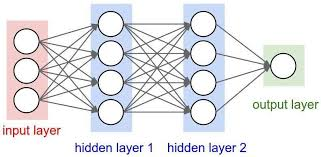多层前馈神经网络可以看作是非线性复合函数，它的学习过程就是将输入沿着网络结构的方向一层，一层的正向传播直到输出层，然后再通过反向传播算法 (BP) 对权重和偏置项进行跟更新。然后循环整个过程，直到满足最大迭代次数或者在验证集上的错误率变化不大。

当问题是回归问题时，损失函数为均方误差:

当问题是分类问题时，损失函数多为交叉熵，最后一层的激活函数为softmax函数:

, z为上一层神经元的输出

展开全文神经网络 多层前馈神经网络
• 提出了有理式多层前馈神经网络的数学模型, 给出了有理式多层神经网络的学习算法. 就计算复杂度而 言, 有理式神经网络的学习算法与传统的多层神经网络反传播算法是同阶的. 函数逼近应用实例结果表明, 将有理式...
• matlab开发-快速多层前馈神经网络训练。这是一个简单而快速的代码，可以训练任意层数的神经网络。
• 多层前馈神经网络有哪些参数Machine learning is solving such a large number of sophisticated problems today that it seems like magic. But there isn’t any magic in machine learning rather it has a ...

多层前馈神经网络有哪些参数

Machine learning is solving such a large number of sophisticated problems today that it seems like magic. But there isn’t any magic in machine learning rather it has a strong mathematical and statistical foundation.

如今，机器学习正在解决如此众多的复杂问题，似乎就像魔术一样。 但是机器学习没有任何魔力，它具有强大的数学和统计基础。

While trying to understand the important and somewhat difficult concepts of machine learning, we sometimes do not even think about some of the trivial concepts. Maybe you think of those, but I know that I ignore a lot of simple things many a times. The reason is the amazing machine learning and deep learning libraries that have functions and methods to quickly do this for us. 😍

在尝试理解机器学习的重要且有些困难的概念时，我们有时甚至没有考虑一些琐碎的概念。 也许您会想到这些，但是我知道我很多次都忽略了许多简单的事情。 原因是令人惊叹的机器学习和深度学习库，它们具有可为我们快速完成此操作的功能和方法。 😍

One such trivial problem is to find the total number of trainable parameters in a feed-forward neural network by hand. A question that I encountered in one of my exams and confused me with the options provided. This question has also been asked in many different forums by many machine learnimg practitioners. 🙋🏻

一个这样的琐碎问题是用手找到前馈神经网络中可训练参数的总数。 我在一项考试中遇到的一个问题，使我对所提供的选项感到困惑。 许多机器学习实践者也在许多不同的论坛中提出了这个问题。 🙋🏻

The problem discussed in this post is:

这篇文章中讨论的问题是：

# 如何找到前馈神经网络中可训练参数的总数？ (How to find the total number of trainable parameters in a feed-forward neural network?)

You must be wondering why is that even an important one to discuss. It is indeed! The time taken to train a model is dependent on the number of parameters to train so this knowledge can really help us at times.

您一定想知道为什么这甚至是一个重要的话题。 这的确是！ 训练模型所需的时间取决于训练参数的数量，因此这些知识有时确实可以为我们提供帮助。

By looking at a simple network, you can easily count and tell the number of parameters. In the worst case, you can draw the diagram and tell the number of parameters. But what happens when you encounter a question of a neural network with 7 layers and a different number of neurons in each layer, say 8, 10, 12, 15, 15, 12, 6. How would you tell how many parameters are there in all?

通过查看一个简单的网络，您可以轻松地计算并告诉参数数量。 在最坏的情况下，您可以绘制图表并告诉参数数量。 但是，当您遇到一个神经网络的问题，该问题具有7层并且每层中有不同数量的神经元时，比如说8、10、12、15、15、12、6，该怎么办？所有？

Let us together find a mathematical formula to get the count. But before moving to the calculation, let us first understand what a feed-forward neural network is and what characteristics it possesses. This will help us in finding the total number of parameters.

让我们一起找到一个数学公式来获取计数。 但是在进行计算之前，让我们首先了解什么是前馈神经网络以及它具有的特征。 这将帮助我们找到参数的总数。

A feed-forward neural network is the simplest type of artificial neural network where the connections between the perceptrons do not form a cycle.

前馈神经网络是最简单的人工神经网络，其中感知器之间的连接不形成循环。

Despite being the simplest neural network, they are of extreme importance to the machine learning practitioners as they form the basis of many important and advanced applications used today. 🤘

尽管它们是最简单的神经网络，但是它们对于机器学习从业者来说却极为重要，因为它们构成了当今使用的许多重要和高级应用程序的基础。 🤘

Characteristics of a feed-forward neural network:

前馈神经网络的特征：

1. Perceptrons are arranged in layers. The first layer takes in the input and the last layer gives the output. The middle layers are termed as hidden layers as they remain hidden from the external world.

感知器分层排列。 第一层接受输入，最后一层提供输出。 中间层被称为隐藏层，因为它们对外部世界保持隐藏。
2. Each perceptron in a layer is connected to each and every perceptron of the next layer. This is the reason for information flowing constantly from a layer to the next layer and hence the name feed-forward neural network.

一层中的每个感知器都连接到下一层的每个感知器。 这就是信息不断从一层流到下一层的原因，因此也就称为前馈神经网络。
3. There is no connection between the perceptrons of the same layer.

同一层的感知器之间没有连接。
4. There is no backward connection (called a feedback connection) from the current layer to the previous layer.

从当前层到上一层没有反向连接(称为反馈连接)。

Note: A perceptron is the fundamental unit of a neural network that calculates the weighted sum of the input values.

注意：感知器是计算输入值的加权和的神经网络的基本单位。

Mathematically, a feed-forward neural network defines a mapping y = f(x; θ) and learns the value of the parameters θ that helps in finding the best function approximation.

在数学上，前馈神经网络定义了一个映射y = f(x;θ)并学习了有助于寻找最佳函数逼近的参数θ的值。

Note: There is also a bias unit in a feed-forward neural network in all the layers except the output layer. Biases are extremely helpful in successful learning by shifting the activation function to the left or to the right. Confused? 🤔 In simple words, bias is similar to an intercept (constant) in a linear equation of the line, y = mx + c, which helps to fit the prediction line to the data better instead of a line always passing through the origin (0,0) (in case of y = mx).

注意：前馈神经网络中除输出层以外的所有层中还存在一个偏置单元。 偏向通过将激活功能向左或向右移动，对于成功学习非常有帮助。 困惑？ simple简单来说，偏差类似于直线的线性方程y = mx + c中的截距(常数)，这有助于使预测线更适合数据，而不是始终穿过原点的线(0 ，0)(如果y = mx)。

Let us now use this knowledge to find the number of parameters.

现在让我们使用此知识来查找参数的数量。

Scenario 1: A feed-forward neural network with just one hidden layer. Number of units in the input, hidden and output layers are respectively 3, 4 and 2.

方案1：仅具有一个隐藏层的前馈神经网络。 输入，隐藏和输出层中的单位数分别为3、4和2。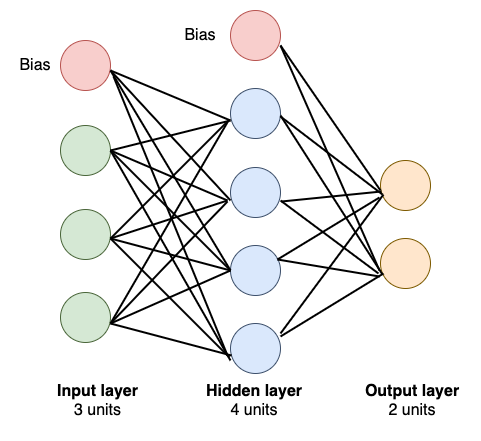A feed-forward neural network (Image by author)

Assumptions:

假设：

i = number of neurons in input layer

i =输入层中神经元的数量

h = number of neurons in hidden layer

h =隐藏层中神经元的数量

o = number of neurons in output layer

o =输出层中神经元的数量

From the diagram, we have i = 3, h = 4 and o = 2. Note that the red colored neuron is the bias for that layer. Each bias of a layer is connected to all the neurons in the next layer except the bias of the next layer.

从图中，我们有i = 3， h = 4和o =2。请注意，红色神经元是该层的偏差。 一层的每个偏置都连接到下一层的所有神经元，除了下一层的偏置。

Mathematically:

数学上：

1. Number of connections between the first and second layer: 3 × 4 = 12, which is nothing but the product of i and h.

第一和第二层之间的连接数：3×4 = 12，这不过是ih的乘积。

2. Number of connections between the second and third layer: 4 × 2 = 8, which is nothing but the product of h and o.

第二层和第三层之间的连接数：4×2 = 8，这不过是ho的乘积。

3. There are connections between layers via bias as well. Number of connections between the bias of the first layer and the neurons of the second layer (except bias of the second layer): 1 × 4, which is nothing but h.

层之间也通过偏置连接。 第一层的偏置与第二层的神经元之间的连接数(第二层的偏置除外)：1×4，仅是h

4. Number of connections between the bias of the second layer and the neurons of the third layer: 1 × 2, which is nothing but o.

第二层的偏置与第三层的神经元之间的连接数：1×2，不过是o

Summing up all:

总结所有：

3 × 4 + 4 × 2 + 1 × 4 + 1 × 2

3×4 + 4×2 +1×4 +1×2

= 12 + 8 + 4 + 2

= 12 + 8 + 4 + 2

= 26

= 26

Thus, this feed-forward neural network has 26 connections in all and thus will have 26 trainable parameters.

因此，该前馈神经网络总共具有26个连接，因此将具有26个可训练的参数。

Let us try to generalize using this equation and find a formula.

让我们尝试使用该方程式进行概括并找到一个公式。

3 × 4 + 4 × 2 + 1 × 4 + 1 × 2

3×4 + 4×2 +1×4 +1×2

= 3 × 4 + 4 × 2 + 4 + 2

= 3×4 + 4×2 + 4 + 2

= i × h + h × o + h + o

= i × h + h × o + h + o

Thus, the total number of parameters in a feed-forward neural network with one hidden layer is given by:

因此，具有一个隐藏层的前馈神经网络中的参数总数由下式给出：

(i × h + h × o) + h + o

(i×h + h×o)+ h + o

Since this network is a small network it was also possible to count the connections in the diagram to find the total number. But, what if the number of layers is more? Let us work on one more scenario and see if this formula works or we need an extension to this.

由于该网络是小型网络，因此还可以对图中的连接进行计数以找到总数。 但是，如果层数更多怎么办？ 让我们在另一个场景中工作，看看这个公式是否有效，或者我们需要对此进行扩展。

Scenario 1: A feed-forward neural network with three hidden layers. Number of units in the input, first hidden, second hidden, third hidden and output layers are respectively 3, 5, 6, 4 and 2.

方案1：具有三个隐藏层的前馈神经网络。 输入，第一隐藏，第二隐藏，第三隐藏和输出层中的单元数分别为3、5、6、4和2。

Assumptions:

假设：

i = number of neurons in input layer

i =输入层中神经元的数量

h1 = number of neurons in first hidden layer

h1 =第一隐藏层中的神经元数量

h2 = number of neurons in second hidden layer

h2 =第二个隐藏层中的神经元数量

h3 = number of neurons in third hidden layer

h3 =第三隐藏层中的神经元数量

o = number of neurons in output layer

o =输出层中神经元的数量

1. Number of connections between the first and second layer: 3 × 5 = 15, which is nothing but the product of i and h1.

第一层和第二层之间的连接数：3×5 = 15，这不过是ih1的乘积。

2. Number of connections between the second and third layer: 5 × 6 = 30, which is nothing but the product of h1 and h2.

第二层和第三层之间的连接数：5×6 = 30，仅是h1h2的乘积。

3. Number of connections between the third and fourth layer: 6 × 4 = 24, which is nothing but the product of h2 and h3.

第三层和第四层之间的连接数：6×4 = 24，这不过是h2和h3的乘积。

4. Number of connections between the fourth and fifth layer: 4 × 2= 8, which is nothing but the product of h3 and o.

第四层与第五层之间的连接数：4×2 = 8，这不过是h3o的乘积。

5. Number of connections between the bias of the first layer and the neurons of the second layer (except bias of the second layer): 1 × 5 = 5, which is nothing but h1.

第一层的偏置与第二层的神经元之间的连接数(第二层的偏置除外)：1×5 = 5，仅是h1

6. Number of connections between the bias of the second layer and the neurons of the third layer: 1 × 6 = 6, which is nothing but h2.

第二层的偏置与第三层的神经元之间的连接数：1×6 = 6，这不过是h2

7. Number of connections between the bias of the third layer and the neurons of the fourth layer: 1 × 4 = 4, which is nothing but h3.

第三层的偏置与第四层的神经元之间的连接数：1×4 = 4，这不过是h3

8. Number of connections between the bias of the fourth layer and the neurons of the fifth layer: 1 × 2 = 2, which is nothing but o.

第四层的偏置与第五层的神经元之间的连接数：1×2 = 2，这不过是o

Summing up all:

总结所有：

3 × 5 + 5 × 6 + 6 × 4 + 4 × 2 + 1 × 5 + 1 × 6 + 1 × 4 + 1 × 2

3×5 + 5×6 + 6×4 + 4×2 +1×5 +1×6 +1×4 +1×2

= 15 + 30 + 24 + 8 + 5 + 6 + 4 + 2

= 15 + 30 + 24 + 8 + 5 + 6 + 4 + 2

= 94

= 94

Thus, this feed-forward neural network has 94 connections in all and thus 94 trainable parameters.

因此，该前馈神经网络总共具有94个连接，因此具有94个可训练参数。

Let us try to generalize using this equation and find a formula.

让我们尝试使用该方程式进行概括并找到一个公式。

3 × 5 + 5 × 6 + 6 × 4 + 4 × 2 + 1 × 5 + 1 × 6 + 1 × 4 + 1 × 2

3×5 + 5×6 + 6×4 + 4×2 +1×5 +1×6 +1×4 +1×2

= 3 × 5 + 5 × 6 + 6 × 4 + 4 × 2 + 5 + 6 + 4 + 2

= 3×5 + 5×6 + 6×4 + 4×2 + 5 + 6 + 4 + 2

= i × h1 + h1 × h2 + h2 × h3+ h3 × o + h1 + h2 + h3+ o

= i × h1 + h1 × h2 + h2 × h3 + h3 × o + h1 + h2 + h3 + o

Thus, the total number of parameters in a feed-forward neural network with three hidden layers is given by:

因此，具有三个隐藏层的前馈神经网络中的参数总数由下式给出：

(i × h1 + h1 × h2 + h2 × h3 + h3 × o) + h1 + h2 + h3+ o

(i×h1 + h1×h2 + h2×h3 + h3×o)+ h1 + h2 + h3 + o

## ŤHUS，公式找到可训练参数的总数在具有n个隐藏层的前馈神经网络由下式给出： (Thus, the formula to find the total number of trainable parameters in a feed-forward neural network with n hidden layers is given by:)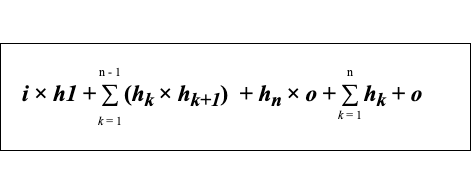Formula to find the total number of parameters in a feed-forward neural network (Image by author)

If this formula sounds a bit overwhelming 😳, don’t worry, there is no need to memorize this formula 🙅. Just keep in mind that in order to find the total number of parameters we need to sum up the following:

如果这个公式听起来有点😳，不要担心，没有必要记住这个公式🙅。 请记住，为了找到参数总数，我们需要总结以下内容：

1. product of the number of neurons in the input layer and first hidden layer

输入层和第一个隐藏层中神经元数量的乘积
2. sum of products of the number of neurons between the two consecutive hidden layers

两个连续的隐藏层之间的神经元数量的乘积之和
3. product of the number of neurons in the last hidden layer and output layer

最后一个隐藏层和输出层中神经元数量的乘积
4. sum of the number of neurons in all the hidden layers and output layer

所有隐藏层和输出层中神经元数量的总和

Now, I hope you can apply this method to find the total number of parameters in a feed-forward neural network with any number of hidden layers and neurons even without using the library. 🙃

现在，我希望您可以使用此方法来查找前馈神经网络中具有任意数量的隐藏层和神经元的参数总数，即使不使用该库也是如此。 🙃

Reference:

参考：

https://cs.stanford.edu/people/eroberts/courses/soco/projects/neural-networks/Architecture/feedforward.html

Thank you! Do leave your comments if you have any feedback or suggestion regarding this post!

谢谢！ 如果您对此帖子有任何反馈或建议，请留下您的评论！

多层前馈神经网络有哪些参数

展开全文神经网络 python java 人工智能
• ## 多层前馈神经网络及BP算法

万次阅读 多人点赞 2018-01-23 14:00:30
1.多层前馈神经网络 首先说下多层前馈神经网络，BP算法，BP神经网络之间的关系。多层前馈（multilayer feed-forward）神经网络由一个输入层、一个或多个隐藏层和一个输出层组成，后向传播(BP)算法在多层前馈神经...

一.多层前馈神经网络
首先说下多层前馈神经网络，BP算法，BP神经网络之间的关系。多层前馈[multilayer feed-forward]神经网络由一个输入层、一个或多个隐藏层和一个输出层组成，后向传播[BP]算法在多层前馈神经网络上面进行学习，采用BP算法的[多层]前馈神经网络被称为BP神经网络。给出一个多层前馈神经网络的拓扑结构，如下所示：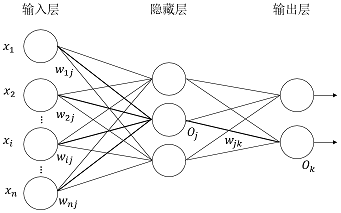神经网络的拓扑结构包括：输入层的单元数、隐藏层数[如果多于一层]、每个隐藏层的单元数和输出层的单元数。神经网络可以用于分类[预测给定元组的类标号]和数值预测[预测连续值输出]等。

二.后向传播[BP]算法详解
1.初始值权重
神经网络的权重被初始化为小随机数，每个神经元都有一个相关联的偏置，同样也被初始化为小随机数。
2.前向传播输入
以单个神经网络单元为例，如下所示：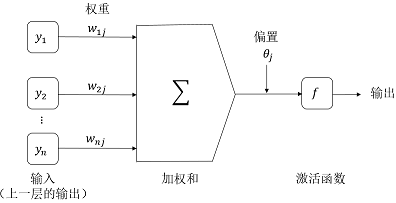给定隐藏层或输出层的单元 j j ，到单元 j j 的净输入 I j I_{j} ，如下所示：
I j = ∑ i w i j O i + θ j {I_j} = \sum\limits_i {{w_{ij}}{O_i} + {\theta _j}}
其中， w i j w_{ij} 是由上一层的单元 i i 到单元 j j 的连接的权重； O i O_i 是上一层的单元 i i 的输出； θ j \theta_j 是单元 j j 的偏置。需要说明的是偏置充当阀值，用来改变单元的活性。
给定单元 j j 的净输入 I j I_j ，单元 j j 的输出 O j O_j ，如下所示：
O j = 1 1 + e − I j {O_j} = \frac{1}{{1 + {e^{ - {I_j}}}}}
3.后向传播误差
对于输出层单元 j j ，误差 E r r j Er{r_j} 用下式计算：
E r r j = O j ( 1 − O j ) ( T j − O j ) Er{r_j} = {O_j}\left( {1 - {O_j}} \right)\left( {{T_j} - {O_j}} \right)
其中， O j O_j 是单元 j j 的实际输出，而 T j T_j j j 给定训练元组的已知目标值。需要说明的是， O j ( 1 − O j ) {O_j}\left( {1 - {O_j}} \right) 是逻辑斯缔函数的导数。
对于隐藏层单元 j j ，它的误差用下式计算：
E r r j = O j ( 1 − O j ) ∑ k E r r k w j k Er{r_j} = {O_j}\left( {1 - {O_j}} \right)\sum\limits_k {Er{r_k}{w_{jk}}}
其中， w j k w_{jk} 是由下一较高层中单元 k k 到单元 j j 的连接权重，而 E r r j Er{r_j} 是单元 k k 的误差。
权重更新，如下所示：
Δ w i j = ( l ) E r r j O i w i j = w i j + Δ w i j \begin{array}{l} \Delta {w_{ij}} = \left( l \right)Er{r_j}{O_i} \\ {w_{ij}} = {w_{ij}} + \Delta {w_{ij}} \\ \end{array}
其中， Δ w i j \Delta {w_{ij}} 是权重 w i j w_{ij} 的改变量，变量 l l 是学习率，通常取0.0和1.0之间的常数值。
偏置更新，如下所示：
Δ θ j = ( l ) E r r j θ j = θ j + Δ θ j \begin{array}{l} \Delta {\theta _j} = \left( l \right)Er{r_j} \\ {\theta _j} = {\theta _j} + \Delta {\theta _j} \\ \end{array}
其中， Δ θ j \Delta {\theta _j} θ j \theta_j 的改变量。
权重和偏置更新
如果每处理一个样本就更新权重和偏置，称为实例更新[case update]；如果处理完训练集中的所有元组之后再更新权重和偏置，称为周期更新[epoch update]。理论上，反向传播算法的数据推导使用周期更新，但是在实践中，实例更新通常产生更加准确的结果。
说明：误差反向传播的过程就是将误差分摊给各层所有单元，从而获得各层单元的误差信号，进而修正各单元的权值，即权值调整的过程。
4.终止条件
如果满足条件之一，就可以停止训练，如下所示：
前一周期所有的都太小，小于某个指定的阀值。
前一周期误分类的元组百分比小于某个阀值。
超过预先指定的周期数。
实践中，权重收敛可能需要数十万个周期。神经网络的训练有很多的经验和技巧，比如可以使用一种称为模拟退火的技术，使神经网络确保收敛到全局最优。

三.用BP训练多层前馈神经网络
举个例子具体说明使用BP算法训练多层前馈神经网络的每个细节，如下所示：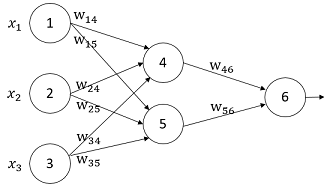设置学习率为0.9，第一个训练元组为 X = { 1 , 0 , 1 } X = \left\{ {1,0,1} \right\} ，其类标号为1。神经网络的初始权重和偏置值如表1所示：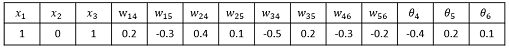根据给定的元组，计算每个神经元的净输入和输出，如表2所示：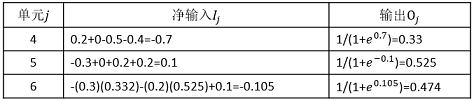每个神经元的误差值如表3所示：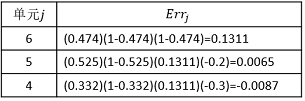说明：从误差的计算过程来理解反向[BP]传播算法也许更加直观和容易。
权重和偏置的更新如表4所示：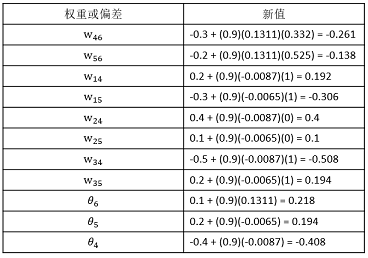说明：将该神经网络模型训练好后，就可以得到权重和偏执参数，进而做二分类。

四.用Python实现BP神经网络
神经网络拓扑结构，如下所示：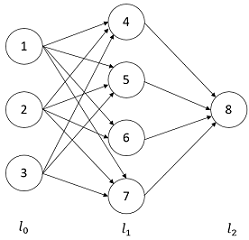解析：
1.第33和35行：l1和l2分别表示第1层和第2层神经元的输出。[第0层表示元组输入]
2.第37行：l2_error与相对应。
3.第40行：l2_delta与输出层误差相对应。
4.第42行：l1_error与相对应。
5.第43行：l1_delta与隐藏层误差相对应。
6.第45行：l1.T.dot(l2_delta)与相对应，而syn1与相对应。
7.第46行：l0.T.dot(l1_delta)与相对应，而syn0与相对应。
说明：一边代码，一边方程，做到代码与方程的映射。这是一个基础的三层BP神经网络，但是麻雀虽小五脏俱全。主要的不足有几点：没有考虑偏置；没有考虑学习率；没有考虑正则化；使用的是周期更新，而不是实例更新[一个样本]和批量更新[m个样本]。但是，足以理解前馈神经网络和BP算法的工作原理。神经网络和BP算法的详细数学推导参考。

参考文献：
数据挖掘：概念与技术[第三版]
使用Python构造神经网络：http://www.ibm.com/developerworks/cn/linux/l-neurnet/
一个11行Python代码实现的神经网络：http://python.jobbole.com/82758/
用BP人工神经网络识别手写数字：http://blog.csdn.net/gzlaiyonghao/article/details/7109898
反向传导算法：http://ufldl.stanford.edu/wiki/index.php/%E5%8F%8D%E5%90%91%E4%BC%A0%E5%AF%BC%E7%AE%97%E6%B3%95

展开全文weight
• 模糊隶属度融合多层前馈神经网络的CBIR方法.pdf
• 提出了一种基于二元蚁群算法的多层前馈神经网络,同时为了避免二元蚁群算法陷入局部最优引入了拥挤交通组织策略。将二元蚁群算法和神经网络混合,可兼有神经网络广泛映射能力和二元蚁群算法快速全局收敛能力,通过在...
• 基于多层前馈神经网络的航天器在线故障检测系统设计.pdf
• 基于多层前馈神经网络SPDS算法的地质数据非线性分析问题研究.pdf
• 基于元胞自动机与多层前馈神经网络的电力信息物理融合系统安全评估模型.pdf
• 多层前馈神经网络善于解决非线性分析问题,但对于复杂的地质数据,普通的训练算法难以收敛。首先介绍了SPDS算法,并把它用于解决地质数据的非线性分析问题。算法的仿真实验表明,用SPDS算法训练的多层前馈神经网络,比较...
• 微信 这是基于MLMVN 代码。 目前它包含 MVN 和 MLMVN 学习的实现。 实现了书中的两个示例： 后函数示例（第 3 章，第 129 页）。 带有 2-2-1 MLMVN 的“三类”示例（第 4 章，第 164 页）。 ...
• 针对目前神经网络在应用中难于确定隐层神经元数的问题,提出了一种神经网络结构的快速修剪算法。该 算法在最优脑外科算法( OBS)的基础上,通过直接剔除冗余的隐层神经元实现神经网络结构自组织设计。实验结果 表明,...
• 由于多层前馈神经网络需要输入值为0附近小范围的数据，因此，我们需要进行一下探索性数据分析，以便于判断是否进行中心化处理。 > summary ( data_csdn ) Y X1 X2 X3 X4 X5 X6 Min . :5 ....
• 在这种结构中，将若干个单层神经网络级联在一起，前一层的输出作为后一层的输入，这样构成了多层前馈神经网络（ Multi-layer Feedforward Neural Networks ）。 更确切地说，每一层神经元仅与下一层的神经元全连接...
• 神经网络灵敏度分析对网络结构设计、硬件实现等具有重要的指导意义，已有的灵敏度计算公式对权值和输入扰动有一定限制或者计算误差较大。基于Piché的随机模型，通过使用两个逼近函数对神经网络一类Sigmoid激活函数...
•神经网络 机器学习
• 一种多层前馈神经网络的快速修剪算法，对网络的修剪有所帮助。神经网络
• 因为公式都是在mathtype上写的，然后插入到ppt的，而且里面有很多图，再用MD写一遍简直太耗时间，所以我就直接当作图片插入发博客了，还请读者谅解。本来ppt是我们每周开组会用的，相互讲解交流，自己做这些也废了...神经网络
• 前馈神经网络（反向传播算法）用到的数据集，包含5000张数字图片X及对应标签y。由于是matlab类型的数据，X需要转置。
• 本节通过设计包含输入层、一个隐含层和输出层的前馈神经网络，实现XOR运算，并基于计算数据或实验数据分析隐含层决策面的特点，进而总结前馈神经网络隐含层的作用。1.1 神经网络结构设计 1.1.1 XOR问题描述 异或是对...神经网络 异或
•神经网络 机器学习
• Python tensorflow 深度前馈神经网络tensorflow
• 1.多层前馈神经网络 首先说下多层前馈神经网络，BP算法，BP神经网络之间的关系。多层前馈（multilayer feed-forward）神经网络由一个输入层、一个或多个隐藏层和一个输出层组成，后向传播(BP)算法在多层前馈神经...
• 神经网络 深度学习： **定义：**深度学习是机器学习的一个研究方向，它基于一种特殊的学习机制。其特点是建立一个多层学习模型，深层级将浅层级的输出作为输入，将数据层层转化，使之越来越抽象。是模拟人脑接受外界...神经网络
• 1）前馈神经网络 (feedforward neural network)，又称作深度前馈网络(deep feedforward network)、多层感知机(multilayer perceptron，MLP) 顾名思义，该网络中没有反向传播反馈，因此无法自动修改网络参数。 2）全...神经网络...